Theory of Gaussian Mixture models¶

In this part are detailed the equations used in each algorithm. We use the same notations as Bishop’s Pattern Recognition and Machine Learning.

Features:

•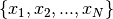is the set of points

Parameters:

•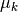is the center of the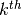cluster
•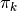is the weight of thecluster
•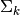is the covariance matrix of thecluster
•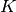is the number of clusters
•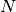is the number of points
•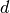is the dimension of the problem

Other notations specific to the methods will be introduced later.

K-means¶

An iteration of K-means includes:

• The E step : a label is assigned to each point (hard assignement) arcording to the means.
• The M step : means are computed according to the parameters.
• The computation of the convergence criterion : the algorithm uses the distortion as described below.

E step¶

The algorithm produces a matrix of responsibilities according to the following equation: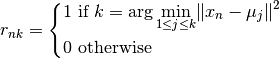The value of the case at the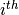row and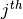column is 1 if thepoint belongs to thecluster and 0 otherwise.

M step¶

The mean of a cluster is simply the mean of all the points belonging to this latter: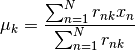The weight of the cluster k, which is the number of points belonging to this latter, can be expressed as: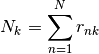The mixing coefficients, which represent the proportion of points in a cluster, can be expressed as: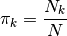Convergence criterion¶

The convergence criterion is the distortion defined as the sum of the norms of the difference between each point and the mean of the cluster it is belonging to: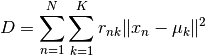The distortion should only decrease during the execution of the algorithm. The model stops when the difference between the value of the convergence criterion at the previous iteration and the current iteration is less or equal to a threshold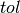: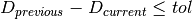Gaussian Mixture Model (GMM)¶

An iteration of GMM includes:

• The E step :probabilities of belonging to each cluster are assigned to each point
• The M step : weights, means and covariances are computed according to the parameters.
• The computation of the convergence criterion : the algorithm uses the loglikelihood as described below.

E step¶

The algorithm produces a matrix of responsibilities according to the following equation: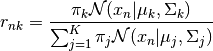The value of the case at therow andcolumn is the probability that the point i belongs to the cluster j.

M step¶

The weight of the cluster k, which is the number of points belonging to this latter, can be expressed as:The mixing coefficients, which represent the proportion of points in a cluster, can be expressed as:As in the Kmeans algorithm, the mean of a cluster is the mean of all the points belonging to this latter: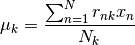The covariance of the cluster k can be expressed as: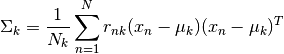These results have been obtained by derivating the maximum loglikelihood described in the following section.

Convergence criterion¶

The convergence criterion used in the Gaussian Mixture Model algorithm is the maximum log likelihood: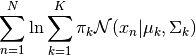Setting its derivatives to 0 gives the empirical terms described in the M step.

Variational Gaussian Mixture Model (VBGMM)¶

In this model, we introduce three new hyperparameters and two distributions which governs the three essential parameters of the model: the mixing coefficients, the means and the covariances.

The mixing coefficients are generated with a Dirichlet Distribution: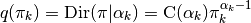The computation of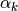is described in the M step.

Then we introduce an independant Gaussian-Wishart law governing the mean and precision of each gaussian component: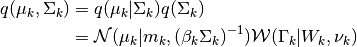The computation of the terms involved in this equation are described in the M step.